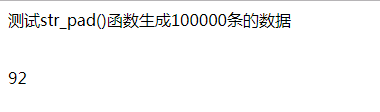# php获取当前时间的毫秒数，并且利用它测试代码段执行时间

87 篇文章 1 订阅

众所周知，php的函数中，有获取当前秒数的time()，也有获取当前微秒数的microtime()，但是并没有获取毫秒数的函数。一般来说都是要我们自己转换的。这边记录一下。

## 一、获取php毫秒数的代码

/*
* php输入毫秒部分的代码
* */
function msectime() {
list($msec,$sec) = explode(' ', microtime());
$msectime = (float)sprintf('%.0f', (floatval($msec) + floatval($sec)) * 1000); return$msectime;
}


1511507346  758
10位的时间戳+3位的毫秒


## 二、以上代码用到的部分

（1）php的microtime（）函数

（2）php的list（）函数

<?php
$my_array = array("Dog","Cat","Horse"); list($a, $b,$c) = $my_array; echo "I have several animals, a$a, a $b and a$c.";

//输出结果
I have several animals, a Dog, a Cat and a Horse.
?>


获取毫秒数的代码里面，相当于用list把通过microtime()获取的毫秒数和时间戳赋给了新的变量， m s e c 是 微 秒 部 分 。 msec是微秒部分。 sec是时间戳部分

（3）php的sprintf()自动填充函数

http://blog.csdn.net/LJFPHP/article/details/78624821

 $msectime = (float)sprintf('%.0f', (floatval($msec) + floatval($sec)) * 1000); //在这句中，'%.0f'中，'%'代表替换符。'f'代表浮点型。'.0'代表小数点位数为0，也就是没有小数点  ## 三、利用获取毫秒的函数测试代码速度：  public function test_iot(Request$request){
//生成10万条数据
$num = 100000;$device_name = 'ABCD_';
$m = 0;$strs = '';
//调用获取毫秒时间的函数，t1
$t1 =$this->msectime();
for($i = 0;$i<$num;$i++){
$m =$m+1;
$str=$device_name.str_pad($m,7,'0',STR_PAD_LEFT );$strs .= '&'.$str; } //生成产品编号后，在此获取当前毫秒时间$t2 = $this->msectime();$strs = substr($strs,1); echo'<p>测试str_pad()函数生成100000条的数据</p>'; //两次时间减一下$t = $t2 -$t1;
echo '<br/>';
print_r($t); // print_r($strs);

}end

06-01
06-02126203-0970
03-27114
12-0649
01-073105
03-0920
03-08515
03-0946
03-10278
09-0852
03-0928
09-111485
01-08411
03-2040
05-07493
01-101010
09-15974
01-111414
04-091万+点击重新获取扫码支付余额充值